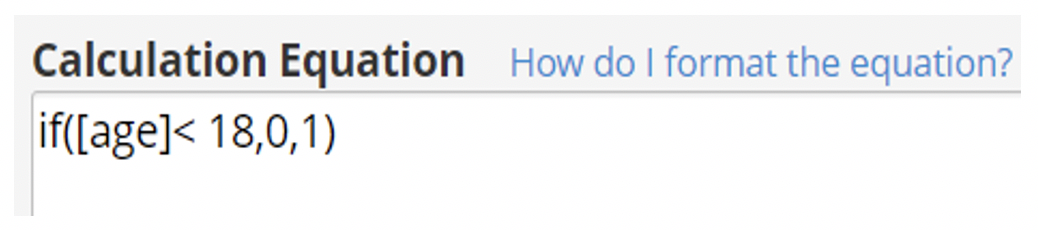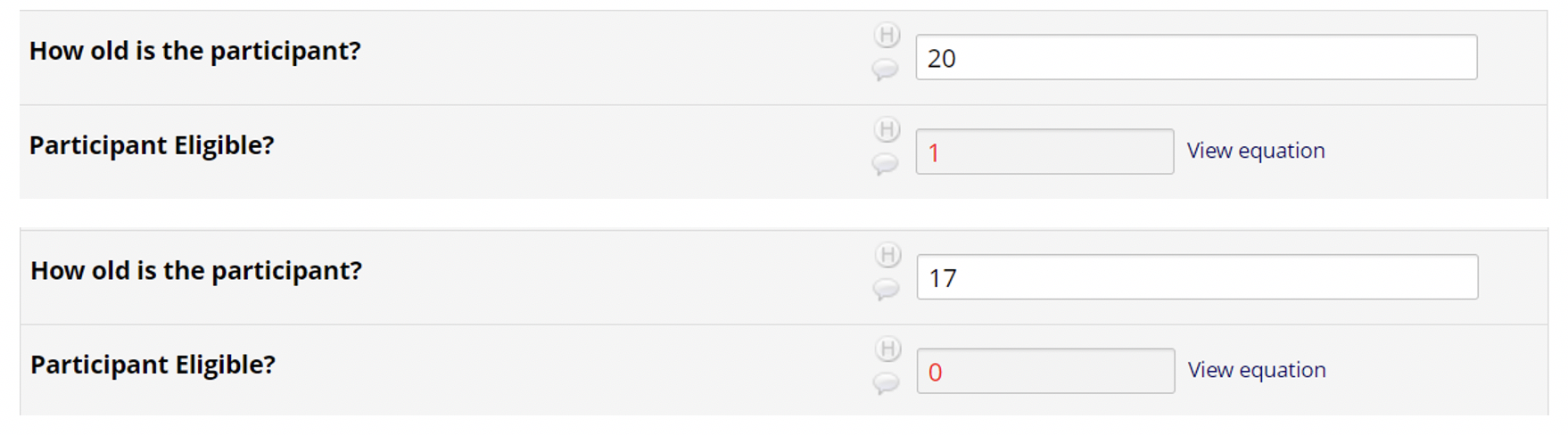# REDCap Tip of the Month: Calculated Fields - If/Then Conditional Logic

The REDCap Team is back with the tips and tricks to keep your May data collection flowering (even if April wasn't rainy).

May 3, 2021

REDCap calculated fields allow for real-time calculations on data entry forms and surveys. REDCap supports addition, subtraction, division and multiplication mathematical operations within a calculated field.

If/Then conditional logic, which you may recognize from Excel, provides the appropriate value based on a conditional field being either true or false. This can be useful for projects wanting to easily

### How do I format the equation?

To add a calculated field, select the “Calculated Field” field type from within the Online Designer. In the “Calculation Equation” textbox, enter the equation for your calculated field. Complete the field label and variable name fields as you normally would.

The equation for an If/Then/Else calculated field looks like this:

if (condition, VALUE if condition is true, VALUE if condition is false).

The condition includes the specific variable along with “=”, “<”, or “>”. Calculated fields in REDCap can only return number values so the value of the condition being true or false must be a number.

### Example:

In the example below, the determination is if a participant is eligible for a study based on their age. As a reminder, REDCap can only return number values so instead of returning “Yes” or “No”, we are formatting the equation to return “1” or “0” where “1” = yes and “0” = no. The equation will be as follows:When completing the data entry form, the “Participant Eligible” field will automatically calculate as soon as the participant age is entered.Implementing an If/Then equation provides the project team with an efficient way to determine conditional results based on data that has been entered into a REDCap project.

For more information regarding Calculated Fields, please click, “How do I format the equation?” located in the calculated field.

## Questions?

Reach out to the REDCap team at ctsiredcap@wakehealth.edu with questions, or submit a REDCap service request via the CTSI Service Request Form.